# Table of derivatives

## The differentiation formula

### Elementary functions

1. The derivative of the number
2.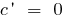3. The derivative of x
4.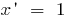5. The derivative degree
6.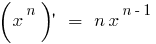7. Derivative of root
8.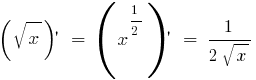9. The derivative of a fraction
10.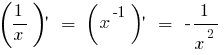11. Derivative e
12.13. The derivative of the number of degree
14.15. Derivative of the natural logarithm
16.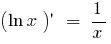17. The derivative of the logarithm
18.19. Derivative of sine
20.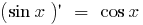21. Derivative of cosine
22.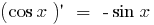23. Derivative of tangent
24.25. Derivative of cotangent
26.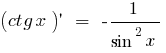27. The derivative of the inverse sine
28.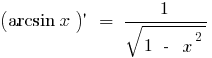29. The arc cosine, the derivative of
30.31. The derivative of the arctangent
32.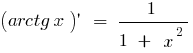33. Derivative of arc cotangent
34.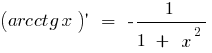### The derivative of the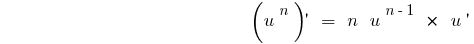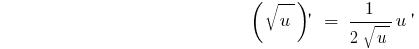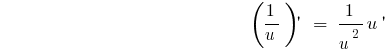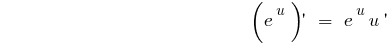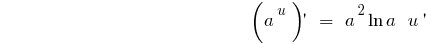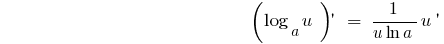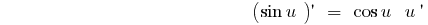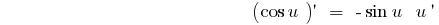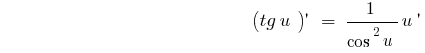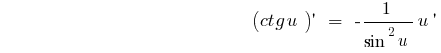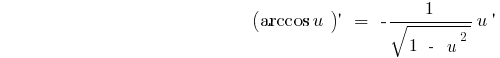## Rules of differentiation

### The derivative of a constant number### The derivative of the sum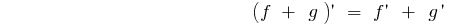### Derivative works### The derivative fraction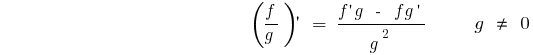### Derivative of a composite function (function of function)

If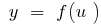and, i.e.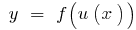,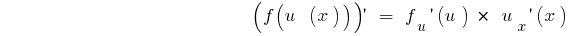Tags:
Chapter:
Versions in other languages:
Share with friends: